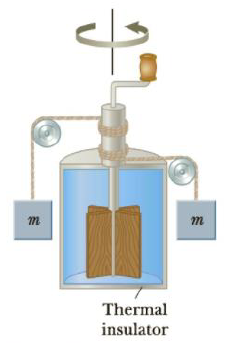Chapter 11, Problem 12P

Chapter
Section
Textbook Problem

The apparatus shown in Figure P11.12 was used by Joule to measure the mechanical equivalent of heat. Work is done on the water by a rotating paddle wheel, which is driven by two blocks falling at a constant speed. The temperature of the stirred water increases due to the friction between the water and the paddles. If the energy lost in the bearings and through the walls is neglected, then the loss in potential energy associated with the blocks equals the work done by the paddle wheel on the water. If each block has a mass of 1.50 kg and the insulated tank is filled with 0.200 kg of water, what is the increase in temperature of the water after the blocks fall through a distance of 3.00 m?Figure P11.12 The falling weights rotate the paddles, causing the temperature of the water to increase.

To determine
How much the increase in the temperature of the water after the blocks fall through a distance.

Explanation

Given Info: Mass of the block is 1.50 kg, mass of the water is 0.200 kg, and the distance the block fall is 3.00 m.

Formula to the change in potential energy of the blocks is,

PE=2mBgh

• PE is the change in potential energy of the block,
• mb is the mass of the block,
• g is the acceleration due to gravity,
• h is the distance the block fell,

The 2 in the above equation is due to two masses.

Formula to calculate the increase in internal energy of the system is,

Q=mWcΔT

Q is the increase in internal energy of the system,

mW is the mass of the water,

c is the specific heat of the water,

ΔT is increase in the temperature,

The change in internal energy of the block is added to the internal energy of the system.

Formula to calculate the change in internal energy of the system is,

Q=PE

Use mWcΔT for Q and 2mBgh for PE in the above equation and rearrange it in terms of ΔT .

mWcΔT=2mBghΔT=2mBghmWc

Substitute 1

Still sussing out bartleby?

Check out a sample textbook solution.

See a sample solution

The Solution to Your Study Problems

Bartleby provides explanations to thousands of textbook problems written by our experts, many with advanced degrees!

Get Started

What is the most common type of star?

Horizons: Exploring the Universe (MindTap Course List)

What is the monomer of a nucleic acid macromolecule?

Biology: The Dynamic Science (MindTap Course List)

What is the major component in gallstones?

Chemistry for Today: General, Organic, and Biochemistry

What is population genetics?

Human Heredity: Principles and Issues (MindTap Course List)

List the four distinguishing chordate traits.

Biology: The Unity and Diversity of Life (MindTap Course List)